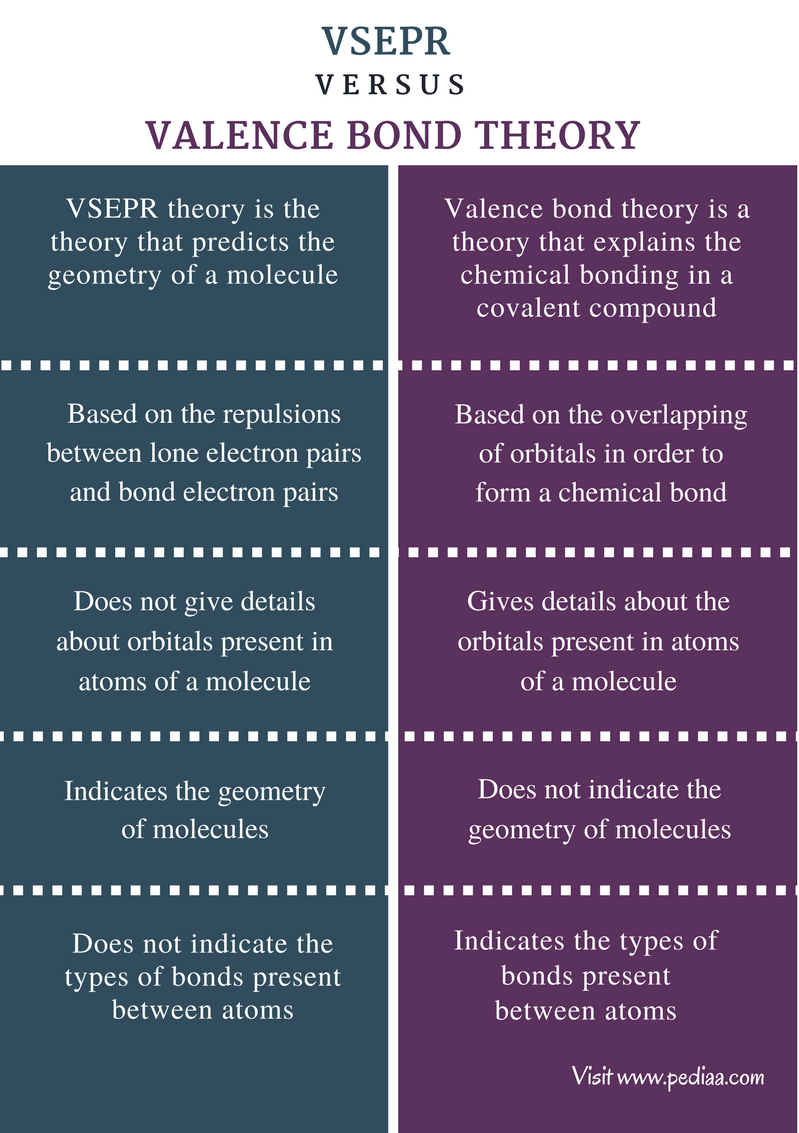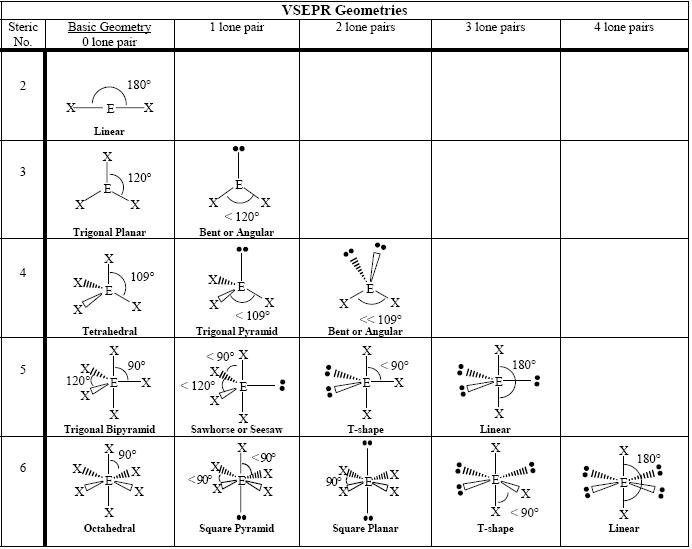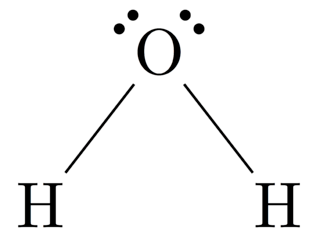# Difference Between VSEPR and Valence Bond Theory

## Main Difference – VSEPR vs Valence Bond Theory

VSEPR and valence bond theory are two theories in chemistry that are used to explain properties of covalent compounds. The VSEPR theory explains the spatial arrangement of atoms in a molecule. This theory uses the repulsions between lone electron pairs and bond electron pairs in order to predict the shape of a certain molecule. The valence bond theory explains the chemical bonding between atoms. This theory explains the overlapping of orbitals in order to form either a sigma bond or a pi bond.  The main difference between VSEPR and valence bond theory is that VSEPR describes the geometry of a molecule whereas valence bend theory describes the chemical bonding in molecules.

### Key Areas Covered

1. What is VSEPR Theory
– Definition, Explanation, Application with Examples
2. What is Valence Bond Theory
– Definition, Explanation, Application with Examples
3. What is the Difference Between VSEPR and Valence Bond Theory
– Comparison of Key Differences

Key Terms: Covalent Bond, Geometry, Hybridization, Pi Bond, Sigma Bond, Valence Bond Theory, VSEPR Theory## What is VSEPR Theory

VSEPR or Valence Shell Electron Pair Repulsion theory is the theory that predicts the geometry of a molecule. Using VSEPR theory, we can propose spatial arrangements for molecules having covalent bonds or coordination bonds. This theory is based on the repulsions between electron pairs in the valence shell of atoms. Electron pairs are found in two types as bond pairs and lone pairs. There are three types of repulsion present in between these electron pairs.

• Bond Pair – bond pair repulsion
• Bond Pair – lone pair repulsion
• Lone Pair – lone pair repulsion

These repulsions occur because all these pairs are electron pairs; since they are all negatively charged, they repel each other. It is important to note that these repulsions are not equal. The repulsion created by a lone pair is higher than that of a bond pair. In other words, lone pairs need more space than bond pairs.

• Repulsion by Lone Pair >  Repulsion by Bond Pair

VSEPR theory can be used to predict both electron geometry and molecular geometry. The electron geometry is the shape of the molecule including the lone pairs present. The molecular geometry is the shape of the molecule considering only the bond electron pairs.

Following shapes are the basic shapes of molecules that can be obtained using the VSEPR theory.Figure 1: Table of Molecular Geometry

The geometry of a molecule is determined by the number of bond pairs and lone pairs around a central atom. The central atom is often the least electronegative atom among other atoms present in the molecule. However, the most precise method to determine the central atom is to calculate the relative electronegativity of each atom. Let us consider two examples.

• BeCl2 (Beryllium Chloride)

The central atom is Be.
It has 2 valence electrons.
Cl atom can share one electron per atom.
Therefore, the total number of electrons around the central atom  = 2 (from Be) + 1×2 (from cl atoms) = 4
Therefore, the number of electron pairs around the Be atom           =  4 / 2    =    2
Number of single bonds present                                                              =  2
Number of lone pairs present                                                                   = 2 – 2     =    0
Therefore, the geometry of BeCl2 molecule is linear.Figure 2: Linear Structure of BeCl2 Molecule

• H2O Molecule

The central atom is O.
Number of valence electrons around O is 6.
Number of electrons shared by H per one atom is 1.
Therefore, the total number of electrons around O     =   6 (O) +  1 x 2 (H) = 8
Number of electron pairs around O                                =   8 / 2 =  4
Number of lone pairs present around O                         =    2
Number of single bonds present around O                    =   2
Therefore, the geometry of H2O is angular.Figure 3: Geometry of H2O Molecule

When looking at the above two examples, both molecules are composed of 3 atoms. Both molecules have 2 single covalent bonds. But the geometries are different from each other. The reason is that H2O has 2 lone pairs but BeCl2 has no lone pairs. The lone pairs on O atom repels the bond electron pairs. This repulsion causes the two bonds to come close to each other. But due to the repulsion between two bond pairs, they cannot come very close. That means, there is a net repulsion between electron pairs around the O atom. This results in an angular shaped molecule rather than a linear one. In BeCl2 molecule, no repulsions occur due to lone pairs since there are no lone pairs. Therefore, only the repulsions of bond pairs occur and the bonds are in the farthest positions where a minimum repulsion occurs.

## What is Valence Bond Theory

Valence bond theory is a theory that explains the chemical bonding in a covalent compound. Covalent compounds are composed of atoms that are bonded to each other through covalent bonds. A covalent bond is a type of chemical bond formed due to the sharing of electrons between two atoms. These atoms share electrons in order to fill their orbitals and become stable. If there are unpaired electrons in an atom it is less stable than an atom having paired electrons. Therefore, atoms form covalent bonds in order to pair all electrons.

Atoms have electrons in their shells. These shells are composed of sub-shells such as s, p, d, etc. Except for s sub-shell, other sub-shell are composed of orbitals. The number of orbitals in each sub-shell are shown below.

 Sub-shell Number of orbitals Names of orbitals s 0 – p 3 px, py, pz d 5 dxz, dxy, dyz, dx2y2, dz2

Each orbital can hold a maximum of two electrons which have opposite spins. The valence bond theory indicates that electron sharing is occurred through overlapping of orbitals. Since electrons are attracted to the nucleus, electrons cannot completely leave the atom. Therefore, these electrons are shared between the two atoms.

There are two types of covalent bonds known as sigma bonds and pi bonds. These bonds are formed due to the overlapping or the hybridization of orbitals. After this hybridization, a new orbital is formed between two atoms. The new orbital is named according to the type of hybridization. A sigma bond is always formed due to the overlapping of two s orbitals. A pi bond is formed when two p orbitals are overlapped.

But when s orbital overlaps a p orbital, it is different from s-s orbital overlapping and p-p orbital overlapping. In order to explain this type of bonding, hybridization of orbitals was found by the scientist Linus Pauling. Hybridization causes the formation of hybrid orbitals. There are three major types of hybrid orbitals as follows.

### sp3 Hybrid Orbitals

This orbital is formed when an s orbital and 3 p orbitals are hybridized. (S orbitals are spherical in shape and p orbitals have a dumbbell shape. The sp3 orbital gets a new shape.) Therefore, the atom now has 4 hybrid orbitals.

### sp2 Hybrid Orbitals

This orbital is formed when an s orbital and 2 p orbitals are hybridized. The shape is different from that of s orbital and p orbitals. The atom now has 3 hybrid orbitals and a non-hybridized p orbital.

### sp Hybrid Orbitals

This orbital is formed when an s orbital and a p orbital are hybridized. The shape is different from that of s orbital and p orbitals. Now the atom has 2 hybrid orbitals and 2 non-hybridized p orbitals.Figure 04: Shapes of Hybrid Orbitals

## Difference Between VSEPR and Valence Bond Theory

### Definition

VSEPR: VSEPR theory is the theory that predicts the geometry of a molecule.

Valence Bond Theory: Valence bond theory is a theory that explains the chemical bonding in a covalent compound.

### Basis

VSEPR: VSEPR theory is based on the repulsions between lone electron pairs and bond electron pairs.

Valence Bond Theory: Valence bond theory is based on the overlapping of orbitals in order to form a chemical bond.

### Orbitals

VSEPR: VSEPR theory does not give details about orbitals present in atoms of a molecule.

Valence Bond Theory: Valence bond theory gives details about the orbitals present in atoms of a molecule.

### Geometry

VSEPR: VSEPR theory gives the geometry of molecules.

Valence Bond Theory: Valence bond theory does not give the geometry of molecules.

### Chemical Bonding

VSEPR: VSEPR theory does not indicate the types of bonds present between atoms.

Valence Bond Theory: Valence bond theory indicates the types of bonds present between atoms.

### Conclusion

Both VSEPR theory and valence bond theory are basic theories that have been developed in order to understand the shapes and bonding of chemical species. These theories are applied to compounds having covalent bonds. The difference between VSEPR and valence bond theory is that VSEPR theory explains the shape of a molecule whereas valence bond theory explains the creation of chemical bonds between atoms of a molecule.

##### References:

1. Jessie A. Key and David W. Ball. “Introductory Chemistry- 1st Canadian Edition.” Valence Bond Theory and Hybrid Orbitals | Introductory Chemistry- 1st Canadian Edition. N.p., n.d. Web. Available here. 28 July 2017.
2. “Explanation of Valence Bond Theory – Boundless Open Textbook.” Boundless. 19 Aug. 2016. Web. Available here. 28 July 2017.

##### Image Courtesy:

1. “VSEPR geometries” By Dr. Regina Frey, Washington University in St. Louis – Own work (Public Domain) via Commons Wikimedia
2. “H2O Lewis Structure PNG” By Daviewales – Own work (CC BY-SA 4.0) via Commons Wikimedia
3. “Orbitale orbitali ibridi” (Pubblico dominio) via Commons Wikimedia